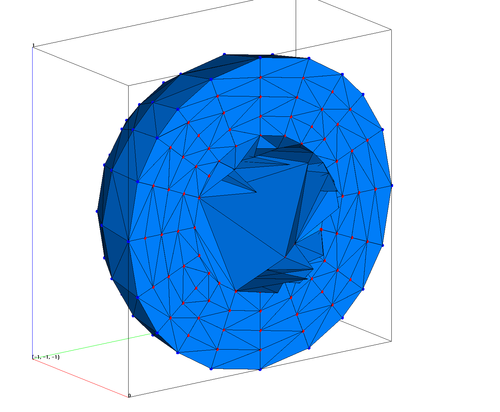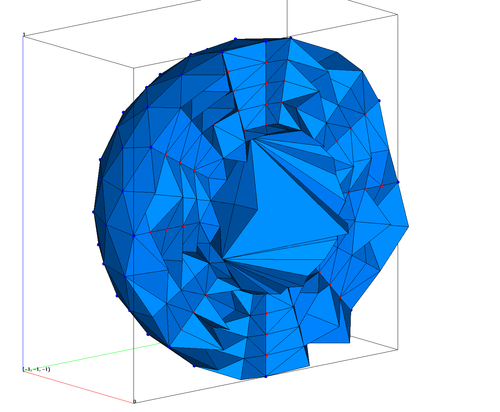CREATEPTS/SPHERE

Builds a sphere by generating coordinates of points and also modifies zoning by ratio-zoning point distributions. See the `rz` command for more details.

FORMAT:

`createpts` /`sphere` / `itype` / `nr,npt,xirad,xorad` / `xcen,ycen,zcen` / `iz` / `irat,rz`

`itype` defines what type or sphere will be created.

• `itype` = 1 generates a sphere by gridding the faces of a cube and then projecting the vertices onto a sphere. The number of nodes per shell is of the form `6*i**2`. Use `connect` to generate tet or triangle connectivity.

• `itype` = 2 generates a sphere by subdividing an icosahedron placed on the surface of a sphere. Icosahedral gridding is made up of 10 diamonds per shell. Each diamond is made up of `n**2` nodes (where n must be of the form `2**i+1`). There are 2 nodes (the poles of the sphere) at which 5 diamonds meet and 10 nodes where 3 diamonds meet; hence there are a minimum of 12 nodes per shell. The number of nodes per shell can be 12, 42, 162, 642,…etc. Use `connect` to generate tet or triangle connectivity.

• `itype` = 8 generates a hexahedral icosahedron grid. This option distributes points and generates the grid connectivity data structures.

• `itype` = `diamond` generates the points for one diamond of the icosahedron

`nr` is the number of radial shells

`npt` is the upper limit of the number of points in a shell, the number of points generated will be less than or equal to this number.

`xirad` , `xorad` are the inner and outer radii of the sphere. For `itype` =8 reverse inner and outer radii.

`xcen`, `ycen`, `zcen` are the coordinates of the center of the sphere

`iz`  if =0 then mins and maxs are used as cell centers, if =1 then mins and maxs are used as cell vertices

`irat` is ratio zoning switch (0=off,1=on)

`rz` is ratio zoning value - distance is multiplied by the value for each subsequent point.

EXAMPLES:

```createpts/sphere/8/5/162/1.0,0.5/0.,0.,0./1,0,0.0/

createpts/sphere/2/5/162/0.5,1.0/0.,0.,0./1,0,0.0/

createpts/sphere/diamond/5/162/1,.5/0,0,0/1,0,0/

```

Projected cube onto a sphere connected into tets (Image clipped at half)
Number of nodes = 750 Number of elements = 4175

```cmo / create / motet_sph
createpts/sphere/1/5/162/1.0,0.5/0.,0.,0./1,0,0.0/
cmo / setatt / motet_sph / imt / 1 0 0 / 1
filter / 1 0 0
rmpoint / compress
connect

```Icosohedron tet sphere connected into tets (Image clipped at half)
Number of nodes = 812 Number of elements = 4187

```cmo / create / motet_sph
createpts/sphere/2/5/162/1.0,0.5/0.,0.,0./1,0,0.0/
cmo / setatt / motet_sph / imt / 1 0 0 / 1
filter / 1 0 0
rmpoint / compress
connect
```